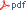﻿ Sébastien BRIOT Webpage

# AMORO: Advanced MOdelling of RObots

#### Master Program

Erasmus Mundus EMARO+ / ARIA-ROBA

#### Semester:

S3 (Master, Second year)

Compulsory

#### Duration:

24 hours + Labs (16 hours)

#### Lecturers:

Stéphane CARO (Part 1: Kinematics - 12 h)

Sébastien BRIOT (Part 2: Dynamics - 12 h)

Sébastien BRIOT

## Objectives of AMORO

This course presents advanced modelling techniques (geometric, kinematic and dynamic) of robots (tree structure robots, parallel robots, and hybrid robots) composed of rigid links.

More details on the general content## AMORO Part 2: Dynamics modelling of complex robot architectures

#### Content:

• Lecture 1: Introduction and recalls (2 hours)
• Introduction to the lecture
• Basics of dynamics principles
• Lagrange formalism (with and without Lagrange multipliers)
• Newton-Euler equations
• Principle of Virtual Powers
• Computation of the link inertial parameters
• Computation of the kinetic and potential energies
• Exercises
• Lecture 2: Dynamics of tree structure robots (2.5 hours)
• Lagrange formalism for open chains (serial and tree-structures)
• Computation of the kinetic and potential energies
• Computation of the robot inertia matrix
• Computation of the Coriolis and centrifugal terms (Christoffels symbols)
• Computation of the gravity vector
• Contributions of the motor inertia and joint friction
• Inverse and direct formulation
• Newton-Euler formalism for open chains (serial and tree-structures)
• Forward recursive computation of velocities and accelerations
• Backward recursive computation of wrenchs
• Contributions of the motor inertia and joint friction
• Computation of the robot inertia matrix and of the Coriolis and centrifugal terms
• Inverse and direct formulation
• Other robot models
• With elasticity in joints
• With closed-loops
• Ground reaction models
• Exercises
• Lecture 3: Computation of the base parameters (1.5 hours)
• Linearity of the dynamics equations and energy equations with respect to the dynamic parameters
• Recursive computation of the energy
• Proof the grouping relations based on the energy functions
• Grouping relations for:
• general revolute joints
• general prismatic joints
• general fixed joints
• Lecture 4: Dynamics of parallel robots (2 hours)
• Recalls on the kinematic contraint relations
• Computation of virtual tree-structure model
• Closing the loops thanks to the Lagrange multipliers
• General form of the dynamics model of parallel robot
• Inverse and direct formulation
• Exercises
• Lecture 5: Analysis of the condition of degeneracy of the dynamic models of parallel robots (2 hours)
• Analysis of the form of the dynamics equations
• Relation between the condition of degeneracy of the dynamic model and the robot singularities
• Condition of non degeneracy of the equations
• Optimal motion planning
• Exercises
• Lecture 6: Introduction to dynamic parameter identification issues (2 hours)
• Linearity of the identification model with respect to the parameters to identify
• Data acquisition and treatment
• Base parameters
• Exciting trajectories
• Statistical analysis
• Non identifiable parameters and essential parametrs
• Examples

## AMORO Labs

#### Labs Content:

• Lab 1: Introduction to ADAMS and ADAMS/Controls (4 hours)
• Introduction to ADAMS
• Creating a mechanism with ADAMS and simulating its behavior in kinematics and dynamics
• Importing CAD files into ADAMS and creating a mechanism
• Introduction to ADAMS/Controls
• Simulating a robot with its controller
• Lab 2: Kinematics of a five-bar mechanism (4 hours)
• Creation of the ADAMS plant
• Geometric analysis
• Velocity analysis
• Acceleration analysis
• Singularity analysis
• Lab 3: Dynamics of a five-bar mechanism (4 hours)
• Creation of the ADAMS plant
• Dynamic analysis
• Condition of degeneracy of the dynamic model
• Planning a trajectory through singularities
• Lab 4: Kinematics and dynamics of a Bi-glide (4 hours - this lab is an exam lab)
• Creation of the ADAMS plant
• Geometric analysis
• Velocity analysis
• Acceleration analysis
• Singularity analysis
• Dynamic analysis
• Condition of degeneracy of the dynamic model
• Planning a trajectory through singularities